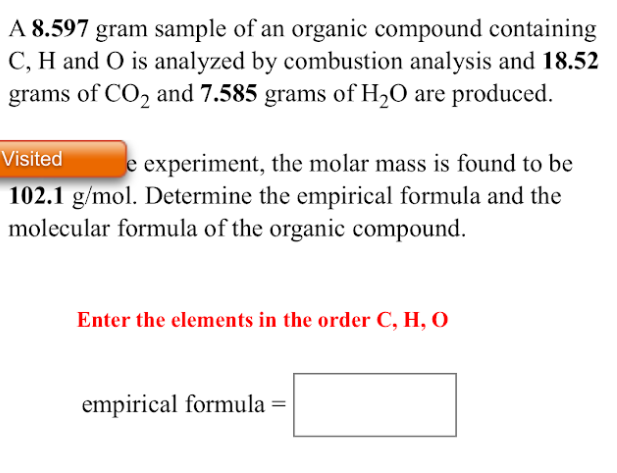# A 8.597 gram sample of an organic compound containing C, H and O is analyzed by combustion analysis and 18.52 grams of CO2 and 7.585 grams of H2O are produced. In a separate experiment, the molar mass is found to be 102.1 g/mol. Determine the empirical formula and the molecular formula of the organic compound. Enter the elements in the order C, H, O empirical formula = ? molecular formula = ? the expri